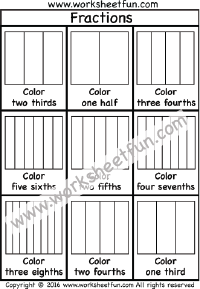Coloring Fractions – Halves, Thirds, Fourths, Fifths, Sixths, Sevenths, Eights – Two Worksheets

Coloring Fractions – Halves, Thirds, Fourths, Fifths, Sixths, Sevenths, EightsMore fraction Circles Worksheets

two thirds, one half, three fourths,five sixths, two fifths, four sevenths, three eighths, two fourths, one third, three sixths, four fifths, two fourths, one third, one half, five sevenths, five eighths, three fourths, two thirds

Fraction

Fraction Circles

Fraction Circles Template

Fraction Model

Fraction Subtraction

Fractions – Coloring

Fractions – Comparing

Fractions – Equivalent